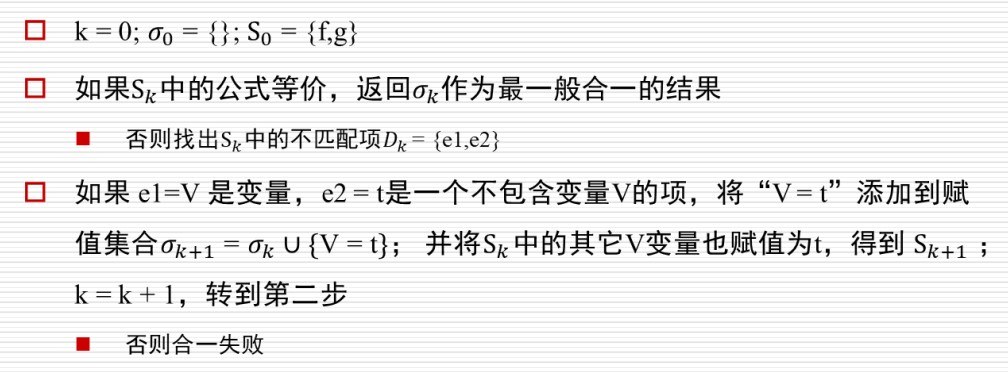# 【人工智能】归结原理 - 用归结算法求解逻辑推理问题

## 基本概念

• 俱乐部成员： tony， mike， john
• 天气类型： rain， snow

• 变量是项（可以看成是0元函数）
• t1， t2， t3……tn是项， f是n元函数，则f(t1,t2,,,,tn)也是项

• 零元谓词：退化为命题
• 单元谓词（unary predicate）：只有一个参数，表示参数具备某种属性，如A(x)表示x属于Aipine俱乐部
• 多元谓词：有多个参数，表示参数之间的关系，如L(x,y)表示x和y具有喜欢关系，即x喜欢y

• S(tony)： tony是skier
• L(tony, rain)： tony喜欢下雨天

• t1， t2， t3……tn是项， P是n元谓词，则P(t1,t2,,,,tn)是原子公式
• t1， t2是项，那么t1=t2是原子公式
• 如果α和β是公式，那么¬α， α˄β， α˅β， ∃α， ∀α都是公式

• 以Aipine俱乐部为例， ∃x(A(x) ∧C(x) ∧¬S(x))是否成立就是在问，是否存在一组实例化（一组赋值），使得A(x) ∧C(x)∧¬S(x)成立，这就是一个可满足性问题。对于该可满足性问题，只要能够找到一组赋值（在这里对应{x}的赋值），使得A(x)∧C(x) ∧¬S(x)成立，那么“A(x) ∧C(x) ∧¬S(x)”是可满足的

• 逻辑蕴含S |= α指对于任意变量赋值，如果S正确，则α也正确
• 逻辑推论S |- α指存在一条推理路径，从S出发，推导证明α

Clausal form：

“合一”是指通过变量替换使得两个子句能够被归结，对应的那一组变量替换，它是一种等价的操作。

## 命题逻辑归结算法

• S |-() 当且仅当 S |= ()， S |= () 当且仅当 S 是不可满足的
• 通过该定理，我们可得KB |= α 当且仅当 KB ∧¬α 不可满足，于是可以通过反证法证明KB |= α

• 将α取否定，加入到KB当中
• 将更新的KB转换为clausal form得到S
• 反复调用单步归结
• • 如果得到空子句，即S|-()，说明KB ∧¬α 不可满足，算法终止，可得KB |= α
• • 如果一直归结直到不产生新的子句，在这个过程中没有得到空子句，则KB |= α不成立

• 从两个子句中分别寻找相同的原子，及其对应的原子否定
• 去掉该原子并将两个子句合为一个，加入到S子句集合中
• 例如(¬child,¬female,girl)和(child)合并为(¬female,girl)

## 最一般合一算法（Most general unifier算法）

• 通过变量替换使得两个子句能够被归结（有相同的原子），所以合一也被定义为使得两个原子公式等价的一组变量替换/赋值
• 由于一阶逻辑中存在变量，所以归结之前需要进行合一，如(P(john),Q(fred),R(x))和(¬P(y),R(susan),R(y))两个子句中，我们无法找到一样的原子及其对应的否定，但是不代表它们不能够归结
• 通过将y替换为john，我们得到了(P(john),Q(fred),R(x))和(¬P(john),R(susan),R(john))，此时我们两个子句分别存在原子P(john)和它的否定¬P(john)，可以进行归结

• 输入：两个原子公式，它们具有相同的谓词，不同的参数项和“¬”
• 输出：一组变量替换/赋值
• 流程• P(f(a),g(x)) 和 P(y,y)无法合一
• P(a,x,h(g(z))) 和 P(z,h(y),h(y))最一般合一为{z=a,x=h(g(a)),y=g(a)}
• P(x,x) 和 P(y,f(y))无法合一

## 一阶逻辑归结算法

• 将α取否定，加入到KB当中
• 将更新的KB转换为clausal form得到S
• 反复调用单步归结
• • 如果得到空子句，即S|-()，说明KB ∧¬α 不可满足，算法终止，可得KB |= α
• • 如果一直归结直到不产生新的子句，在这个过程中没有得到空子句，则KB |= α不成立

• 使用MGU算法从两个子句中得到相同的原子，及其对应的原子否定
• 去掉该原子并将两个子句合为一个，加入到S子句集合中
• 例如(¬Student(x),HardWorker(x))和(HardWorker(sue))合并为(¬Student(sue))

## 【编程】用归结算法求解逻辑推理问题

1. (P(x),Q(g(x)))
2. (R(a),Q(z),¬P(a))
3. R[1a,2c]{X=a} (Q(g(a)),R(a),Q(z))
… …
“R” 表示归结步骤.
“1a” 表示第一个子句(1-th)中的第一个 (a-th)个原子公式，即P(x).
“2c”表示第二个子句(1-th)中的第三个 (c-th)个原子公式，即¬P(a).
“1a”和“2c”是冲突的，所以应用最小合一{X = a}.

On(aa,bb)
On(bb,cc)
Green(aa)
¬Green(cc)
(¬On(x,y),¬Green(x),Green(y))

(¬Student(x),HardWorker(x))
¬HardWorker(sue)

A(tony)
A(mike)
A(john)
L(tony, rain)
L(tony, snow)
(¬A(x), S(x), C(x))
(¬C(y), ¬L(y, rain))
(L(z, snow), ¬S(z))
(¬L(tony, u), ¬L(mike, u))
(L(tony, v), L(mike, v))
(¬A(w), ¬C(w), S(w))